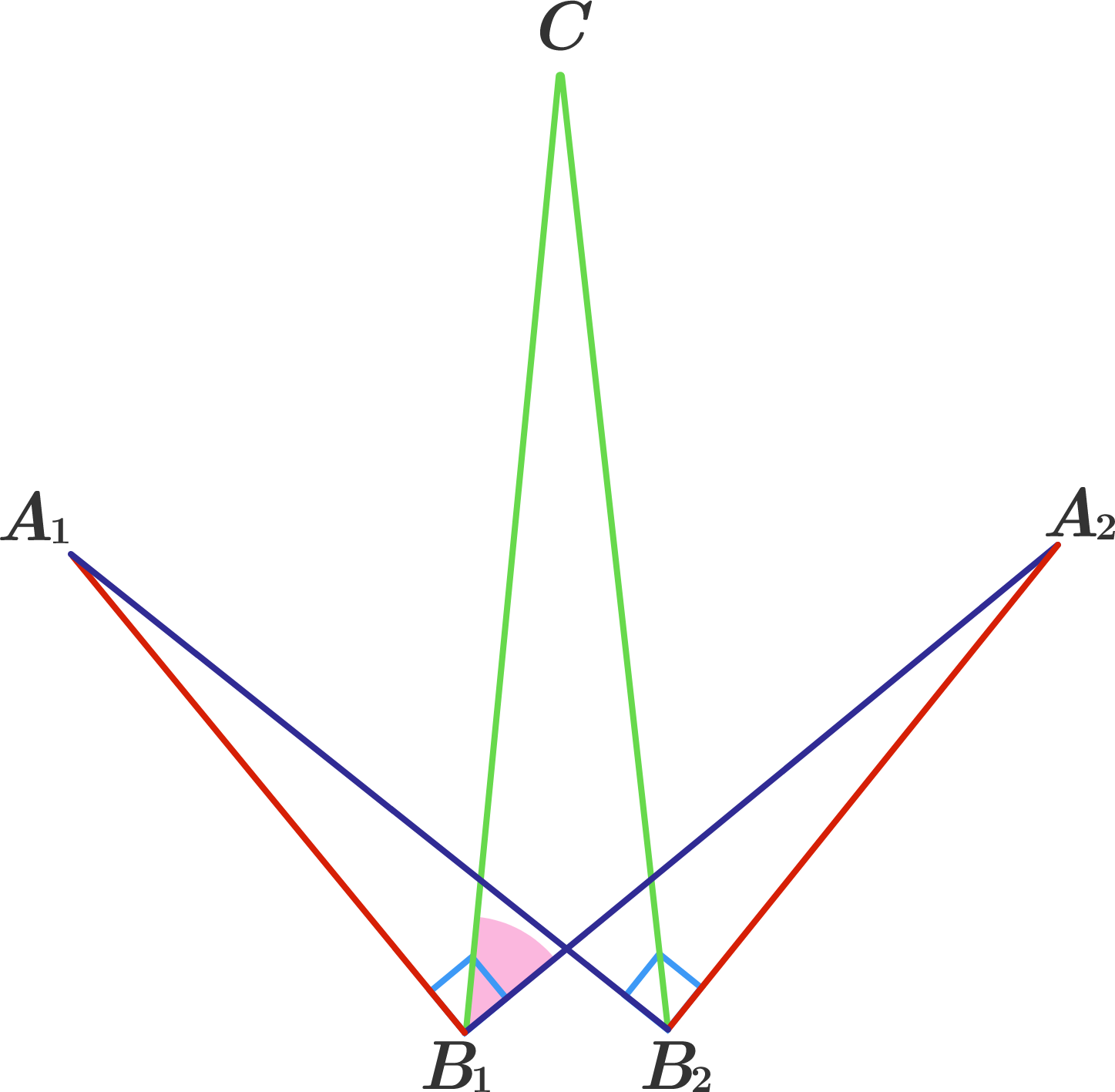# Symmetrical Spiky Polygon

Geometry Level 1The figure to the right consists of 6 straight lines. Suppose the following is known:

• $|\overline{A_1B_1}| = |\overline{A_2B_2}|,\ |\overline{A_1B_2}| = |\overline{A_2B_1}|,\$ and $|\overline{B_1C}| = |\overline{B_2C}|.$
• $\overline{A_1B_1} \perp \overline{A_2B_1}$ and $\overline{A_1B_2} \perp \overline{A_2B_2}.$
• The three acute angles $A_1, A_2,$ and $C$ have congruent measures.

What is the measure of $\angle CB_1A_2?$

×